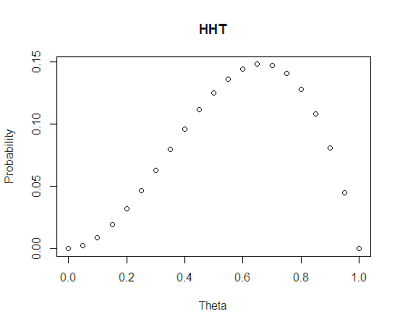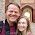## Sunday, September 8, 2013

### Maximum Likelihood Estimation and the Origin of Life

```

# Maximum likelihood Estimation (MLE) is a powerful tool in econometrics and statistics which allows for the consistent and asymptotically efficient estimation of parameters given a correct identification (in terms of distribution) of the random variable.

# It is also a proceedure which seems superficially quite complex and intractible, but in reality is very intuitive and easy to use.

# To introduct MLE, lets think about observing a repeated coin flip.

# Let's say we know it is not a fair coin and we would like to figure out what the likehood of heads parameter popping up is.

# Let's define out likelihood function this way:

lk <- function(responses, theta) {
# This likelihood function returns the likelihood of (a priori) observing a
# particular response pattern given the likelihood of seeing a head on the
# coin is theta.

returner <- 0*theta
# Define a vector to hold likelihoods in case theta is a vector

for (i in 1:length(theta)) returner[i] <-
prod(theta[i]^responses) * # The likelihood of seeing the head's pattern
prod((1-theta[i])^(1-responses)) # The likelihood of seeing the tails's pattern

returner
}

# Let's see how our likelihood function is working.
# Let's see we flip the coin once and we observe a heads
# and our initial guess is that the coin is fair.
lk(c(1), .5)
#  0.5
# Our likelihood of this being true is .5 (we know this is right)

# What is we get a tail?
lk(c(0), .5)
#  0.5
# .5 as well.  This seems right.

# Now let's try something more interesting.
# Let's say we get two heads:
lk(c(1,1), .5)
#  0.25
# .5^2=.25

# This is standard probability. But, let's now ask:
# What if the coin was not fair (as we originally guessed)?

# Is there a better parameter set that would lead to our observed outcome?
# Let's say, there is a 75% chance of getting heads.
# What is the likelihood of seeing our 2 heads.
lk(c(1,1), .75)
#  0.5625
# So if the coin was unfair at 75% then our likelihood of observing the
# outcome we did would increase from 25% to 56%.

# How about is the coin were 95% in favor of heads.
lk(c(1,1), .95)
#  0.9025
# Now we are up to 90%.

# You probably see where this is going.
# If we assume we know nothing about the underlying distribution of the coin
# parameter, then the parameter which fits best is 1 (100%).
# Thus if we see only positive outcomes then the most likely guess at the
# underlying distribution of outcomes is that every time you flip the coin it

# We can see this mapped out.
theta.vect <- seq(0,1,.05)

plot(theta.vect, lk(c(1,1), theta.vect), main="HH",
ylab="Probability", xlab="Theta")# It is worth noting that though there are different probabilities
# for each unfairness parameter. We can only definitively rule out
# one option after flipping the coin twice and getting heads.
# That is that it is impossible for the likelihood of getting a head=0.

# However, all other options are available. How do we choose from these options?

# Well... naturally, we choose the option which maximizes the probability
# of seeing the observed outcome (as the name implies).

# Now let's make things a little more interesting. Let's say the third
# time we flip the coin it ends up tails.

# If we assume it is a fair coin then:
lk(c(1,1,0), .5)
#  0.125

lk(c(1,1,0), .75)
#  0.140625
# More likely than that of a fair coin but not as dramatic an improvement from
# when we saw only two heads.

# Let's see how the graph looks now.
plot(theta.vect, lk(c(1,1,0), theta.vect), main="HHT",
ylab="Probability", xlab="Theta")# We can see that our graph is finally beginning to look a bit more interesting.
# We can see that the most likely outcome is around 65%.  For those of us
# a little ahead of the game the most likely probability is the success rate
# or 2/3 (66.6%).

# But the importance of the exercise to think about why 66.6% is parameter
# we select as the most likely.
lk(c(1,1,0), 2/3)
#  0.1481481
# Not because it is overwhelmingly the best choice.

# It is only 2.3% (0.148-0.125) more likely to occur than if it were a fair coin.
# So we really are not very confident with our parameter choice at this point.

# However, imagine instead for one moment, if we observed the same ratio but with
# 300 coins.
cpattern <- c(rep(1,200), rep(0,100))

lk(cpattern, 2/3)
#  1.173877e-83

lk(cpattern, 1/2)
#  4.909093e-91

# Now, in terms of percentages the differences are extremely small.
# So small that it is hard to compare.  The plot can be useful:

plot(theta.vect, lk(cpattern, theta.vect),
main="(HHT)^100", ylab="Probability", xlab="Theta")# Let's see what happens if we increase our number of coins to
# 3000
plot(theta.vect, lk(rep(cpattern,10), theta.vect),
main="(HHT)^1000", ylab="Probability", xlab="Theta")# In this graph we can see the first major computational problem
# when dealing with likelihoods. They get so small, they are hard
# to manage in raw probabilities.  In this case the digits get
# rounded into 0 so that all R sees is 0.

# Fortunately, the maximum of a function is the same maximum
# (in terms of parameter choices) as a monotonic transformation
# of a function.  Thus we can rescale our probabilities
# before multiplication using logs creating the log likelihood
# function which produces parameters which vary in scale much less
# dramatically.

# MLE functions always maximizes and reports the "log likelihood"
# value rather than the "likelihood".

# However, in this discussion it is worth noting that there is
# a somewhat useful statistic that we can produce to compare
# the likelihoods of the fair coin hypothesis with that
# of the 2/3 biased hypothesis.

# That is the odds ratio of the two outcomes.  How much more
# likely (multiplicatively) is our outcome to be observed
# if the coin is unfair towards 2/3 heads rather than fair?

lk(cpattern, 2/3)/lk(cpattern, 1/2)
#  23912304
# That is to say, the outcome in which 2/3 rds of the time
# we get heads for a coin flip of 300 coins is 23 million
# times more likely to occur if our coin
# is unfair (66.6%) over that of being fair (50%).

# This is a pretty big number and thus very unlikely to occur
# relative to that of a fair coin. Comparing accross all possible
# outcomes, we would find that while this ratio is not always as
# large, for example if we are comparing 2/3s to .6
lk(cpattern, 2/3)/lk(cpattern, .75)
#  183.3873
# But it can still be quite large.  In this case, we are 183 times
# more likely to see the outcome we saw if we chose 2/3s as our parameter
# choice compared with 3/4ths.

# Looking at the raw probability we see
lk(cpattern, 2/3)
#  1.173877e-83
# or 1 out of 8*10^82 outcomes.

# Thus the likelihood of a particular event ever occurring is very small, even
# given the most likely hypothesis (theta=2/3).
# However, compared nearly all other hypothesis such as (theta=1/2 or 3/4)
# the event is much more likely to have occurred.

# And THAT is why creationists are right to say it very unlikely
# in absolute terms that evolution brought about the origin of
# life on earth yet are also completely wrong because compared
# with all other available hypotheses that is the only one
# which is remotely likely (at least from the series of
# outcomes that I have observed) to have occurred makes its odds
# ratio very high in my mind.

Created by Pretty R at inside-R.org```

1.First, equating evolution with the origin of life is incorrect. Evolution is generally defined as heritable changes passed on from primitive organisms to future generations. For evolution to occur, you have to already have an organism that is capable of passing on heritable changes. When discussing "Origin of Life" from a Non-Creationist perspective, what is really meant is Abiogenesis. This is the formation of a living organism from non-living organic compounds. This has NEVER been observed, tested or repeated making it the antithesis of "science" and a creation myth of its own. Where is the supporting evidence from a statistical, scientific stand point that makes Abiogenesis "more likely" than Creationism? I didn't see any.

1.Sara, Abiogenesis, is not what I was referring to. However, as for your point that life has never been replicated in a sterile lab. I am glad you have a standard sufficiently high that it is unlikely to ever be matched in your lifetime. However, just because we have not yet created life does not mean much since the Earth had billions of years and a lab the size of a planet. We will find out better answers to this debate if we ever get outside or solar system.

2.What do you think of this? http://www.sciencedaily.com/releases/2009/01/090109173205.htm

2.A maximum likelihood estimator is a method from statistics. Econometrics is applied mathematics and statistics. Might seem trivial, but to narrow the focus of MLEs to econometrics doesn't do it justice.

1.Very appropriate criticism. I personally see very little difference between statistics and econometrics. Excuse me for any unintended offense.